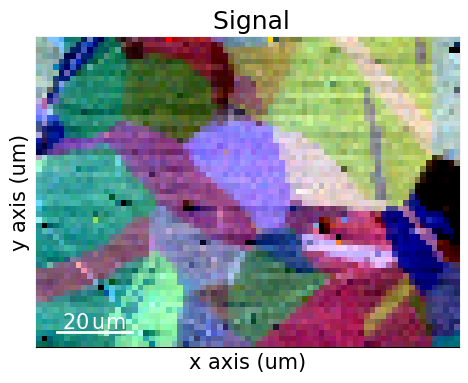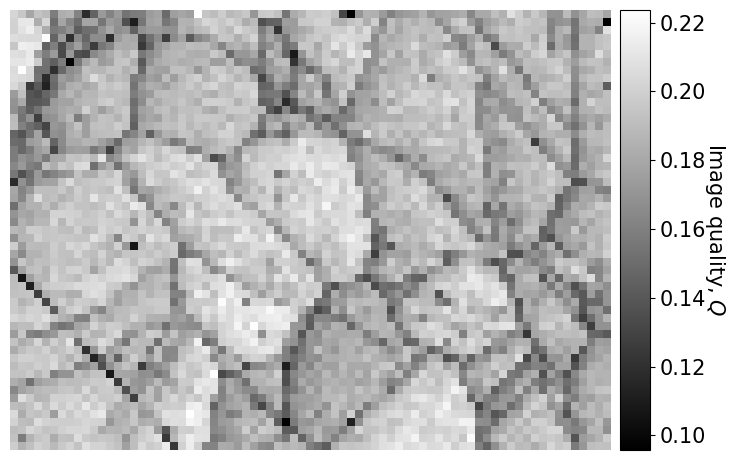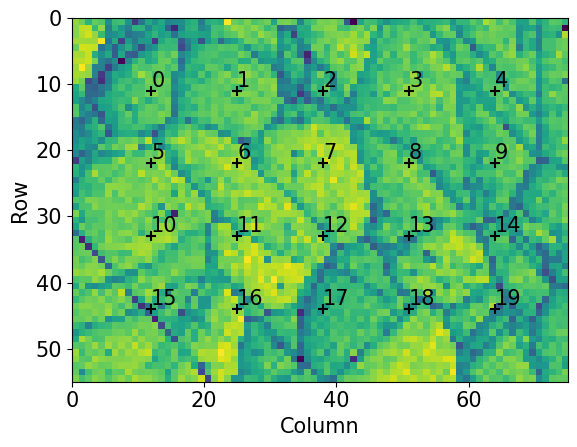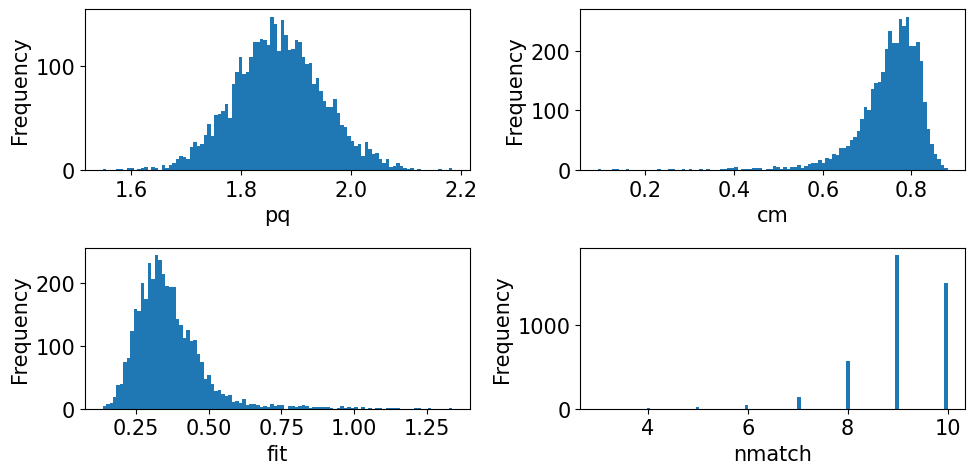# Hough indexing#

In this tutorial, we will perform Hough indexing (HI) using PyEBSDIndex. We will use a tiny 13 MB dataset of nickel available with kikuchipy.

Note

PyEBSDIndex is an optional dependency of kikuchipy, and can be installed with both pip and conda (from conda-forge). To install PyEBSDIndex, see their installation instructions.

PyEBSDIndex supports indexing face centered and body centered cubic (FCC and BCC) materials.

Let’s import necessary libraries

:

# Exchange inline for notebook or qt5 (from pyqt) for interactive plotting
%matplotlib inline

import matplotlib.pyplot as plt
import numpy as np

from diffpy.structure import Atom, Lattice, Structure
from diffsims.crystallography import ReciprocalLatticeVector
import kikuchipy as kp
from orix import plot
from orix.crystal_map import Phase, PhaseList
from orix.vector import Vector3d

plt.rcParams.update(
{"font.size": 15, "lines.markersize": 15, "scatter.edgecolors": "k"}
)


Load a dataset of (75, 55) nickel EBSD patterns of (60, 60) pixels with a step size of 1.5 μm

:

s = kp.data.nickel_ebsd_large(allow_download=True)
s

2023-07-24 16:14:30,544 - hyperspy.extensions - INFO - Enabling extension kikuchipy

:

<EBSD, title: patterns Scan 1, dimensions: (75, 55|60, 60)>


## Pre-indexing maps#

First, we produce two indexing independent maps showing microstructural features: a virtual backscatter electron (VBSE) image and an image quality (IQ) map. The former uses the BSE yield on the detector to give a qualitative orientation contrast, so is done on raw unprocessed patterns. The latter assumes that the sharper the Kikuchi bands, the higher the image quality, so is done on processed patterns.

:

vbse_imager = kp.imaging.VirtualBSEImager(s)
print(vbse_imager.grid_shape)

(5, 5)


Get the VBSE image by coloring the three center grid tiles red, green and blue

:

maps_vbse_rgb = vbse_imager.get_rgb_image(r=(2, 1), g=(2, 2), b=(2, 3))
maps_vbse_rgb

:

<VirtualBSEImage, title: , dimensions: (|75, 55)>


Plot the VBSE image

:

maps_vbse_rgb.plot()We see that we have 20-30 grains, many of them apparently twinned.

Enhance the Kikuchi bands by removing the static and dynamic background (see the pattern processing tutorial for details)

:

s.remove_static_background()
s.remove_dynamic_background()

[########################################] | 100% Completed | 207.96 ms
[########################################] | 100% Completed | 809.90 ms


Get the IQ map

:

maps_iq = s.get_image_quality()

[########################################] | 100% Completed | 408.16 ms


Plot the IQ map (using the CrystalMap.plot() method of the EBSD.xmap attribute)

:

s.xmap.plot(
maps_iq.ravel(),  # Must be 1D
cmap="gray",
colorbar=True,
colorbar_label="Image quality $Q$",
)We recognize the grain and (presumably) twinning boundaries from the VBSE image, and also some dark lines, e.g. to the lower and upper left, which look like scratches on the sample surface.

## Calibrate detector-sample geometry#

We need to know the position of the sample with respect to the detector, the so-called projection/pattern center (PC) (see the reference frames tutorial for all conventions). We do this by optimizing an initial guess of the PC obtained from similar experiments on the same microscope.

We will keep all detector-sample geometry parameters conveniently in an EBSDDetector

:

sig_shape = s.axes_manager.signal_shape[::-1]  # (Rows, columns)
det = kp.detectors.EBSDDetector(sig_shape, sample_tilt=70)

det

:

EBSDDetector (60, 60), px_size 1 um, binning 1, tilt 0, azimuthal 0, pc (0.5, 0.5, 0.5)


Extract patterns from the full dataset spread out evenly in a map grid

:

grid_shape = (5, 4)
s_grid, idx = s.extract_grid(grid_shape, return_indices=True)
s_grid

:

<EBSD, title: patterns Scan 1, dimensions: (5, 4|60, 60)>


Plot the pattern grid on the IQ map

:

nav_shape = s.axes_manager.navigation_shape[::-1]

kp.draw.plot_pattern_positions_in_map(
rc=idx.reshape(2, -1).T,  # Shape (n patterns, 2)
roi_shape=nav_shape,  # Or maps_iq.shape
roi_image=maps_iq,
)We will optimize one PC per pattern in this grid using EBSD.hough_indexing_optimize_pc(), which calls the PyEBSDIndex function pcopt.optimize() internally. Hough indexing with PyEBSDIndex is centered around the use of an EBSDIndexer. The indexer stores the phase and detector information as well as the indexing parameters, like the resolution of the Hough transform and the number of bands to use for orientation determination. Here, we obtain this indexer by combining a PhaseList with an EBSDDetector via EBSDDetector.get_indexer()

:

phase_list = PhaseList(
Phase(
name="ni",
space_group=225,
structure=Structure(
lattice=Lattice(3.5236, 3.5236, 3.5236, 90, 90, 90),
atoms=[Atom("Ni", [0, 0, 0])],
),
),
)
phase_list

:

Id  Name  Space group  Point group  Proper point group     Color
0    ni        Fm-3m         m-3m                 432  tab:blue

:

indexer = det.get_indexer(phase_list)

print(indexer.vendor)
print(indexer.sampleTilt)
print(indexer.camElev)
print(indexer.PC)
print(indexer.phaselist)

KIKUCHIPY
70
0
[0.5 0.5 0.5]
['FCC']


Optimize PCs for each grid pattern using the Nelder-Mead optimization algorithm from SciPy. (We will “overwrite” the existing detector variable.)

:

det = s_grid.hough_indexing_optimize_pc(
pc0=[0.4, 0.2, 0.5],  # Initial guess based on previous experiments
indexer=indexer,
batch=True,
)

# Print mean and standard deviation
print(det.pc_flattened.mean(axis=0))
print(det.pc_flattened.std(0))

[0.4208205  0.21410182 0.50259016]
[0.00983586 0.00674822 0.00451099]


Plot the PCs

:

det.plot_pc("scatter", s=50, annotate=True)The values do not order nicely in the grid they were extracted from… This is not that surprising though, seeing that they are only (60, 60) pixels wide! Fortunately, the spread is not great, so we will can use the mean PC for indexing.

:

det.pc = det.pc_average


We can check the position of the mean PC on the detector before using it

:

det.plot(pattern=s_grid.inav[0, 0].data)## Perform indexing#

With this PC calibration, we can index all patterns. We will get a new indexer from the detector with the average PC as determined from the optimization above

:

indexer = det.get_indexer(phase_list)
indexer.PC

:

array([0.4208205 , 0.21410182, 0.50259016])


Now we are ready to index our patterns using EBSD.hough_indexing(). After indexing is done, we will also plot the Hough transform of the first pattern with the nine detected bands used in indexing highlighted (by passing verbose=2 on top PyEBSDIndex). Although we passed the phase list to create the indexer with EBSDDetector.get_indexer() above, we need to pass it to EBSD.hough_indexing() to obtain describe the phase(s) correctly in the returned CrystalMap

:

xmap = s.hough_indexing(phase_list=phase_list, indexer=indexer, verbose=2)

Hough indexing with PyEBSDIndex information:
PyOpenCL: False
Projection center (Bruker): (0.4208, 0.2141, 0.5026)
Indexing 4125 pattern(s) in 8 chunk(s)
Convolution Time: 3.9881880309985718
Peak ID Time: 3.3646533619939873
Band Label Time: 1.0317313900050067
Total Band Find Time: 14.74000654700285
Band Vote Time:  5.126914713000588
Indexing speed: 207.25273 patterns/s

<Figure size 640x480 with 0 Axes>:

xmap

:

Phase   Orientations  Name  Space group  Point group  Proper point group     Color
0  4125 (100.0%)    ni        Fm-3m         m-3m                 432  tab:blue
Properties: fit, cm, pq, nmatch
Scan unit: um


## Validate indexing results#

Plot quality metrics

:

aspect_ratio = xmap.shape / xmap.shape
figsize = (8 * aspect_ratio, 4.5 * aspect_ratio)

fig, ax = plt.subplots(nrows=2, ncols=2, figsize=figsize)
for a, to_plot in zip(ax.ravel(), ["pq", "cm", "fit", "nmatch"]):
im = a.imshow(xmap.get_map_data(to_plot))
fig.colorbar(im, ax=a, label=to_plot)
a.axis("off")The pattern quality (PQ) and confidence metric (CM) maps show little variation across the sample. The most important map here is the pattern fit (also known as the mean angular error/deviation), which shows the average angular deviation between the positions of each detected band to the closest theoretical band: this is below an OK fit of 1.5$$^{\circ}$$ across most of the map. The final map (nmatch) shows that most of the nine detected bands in each pattern were indexed within a pattern fit of 3$$^{\circ}$$. See the PyEBSDIndex Hough indexing tutorial for a complete explanation of all the indexing result parameters.

Create a color key to color orientations with

:

v_ipf = Vector3d.xvector()
sym = xmap.phases.point_group

ckey = plot.IPFColorKeyTSL(sym, v_ipf)
ckey

:

IPFColorKeyTSL, symmetry: m-3m, direction: [1 0 0]


Orientations are given a color based on which crystal direction $$\left<uvw\right>$$ points in a certain sample direction, producing the so-called inverse pole figure (IPF) map. Let’s plot the IPF-X map with the CM map overlayed

:

rgb_x = ckey.orientation2color(xmap.rotations)
fig = xmap.plot(rgb_x, overlay="cm", remove_padding=True, return_figure=True)

# Place color key in bottom right corner, coordinates are [left, bottom, width, height]
[0.77, 0.07, 0.2, 0.2], projection="ipf", symmetry=sym
)
ax_ckey.plot_ipf_color_key(show_title=False)
ax_ckey.patch.set_facecolor("None")Let’s also plot the three maps side by side

:

directions = Vector3d(((1, 0, 0), (0, 1, 0), (0, 0, 1)))
n = directions.size

figsize = (4 * n * aspect_ratio, n * aspect_ratio)
fig, ax = plt.subplots(ncols=n, figsize=figsize)
for i, title in zip(range(n), ["X", "Y", "Z"]):
ckey.direction = directions[i]
rgb = ckey.orientation2color(xmap.rotations)
ax[i].imshow(rgb.reshape(xmap.shape + (3,)))
ax[i].set_title(f"IPF-{title}")
ax[i].axis("off")The orientation maps show grains and twins as we would expect from the VBSE image and IQ map obtained before indexing.

As a final verification, we’ll plot geometrical simulations on top of the experimental patterns (see the geometrical simulations tutorial for details)

:

rlv = ReciprocalLatticeVector(
phase=xmap.phases, hkl=[[1, 1, 1], [2, 0, 0], [2, 2, 0], [3, 1, 1]]
)
rlv = rlv.symmetrise()
simulator = kp.simulations.KikuchiPatternSimulator(rlv)
sim = simulator.on_detector(det, xmap.rotations.reshape(*xmap.shape))

Finding bands that are in some pattern:
[########################################] | 100% Completed | 103.59 ms
Finding zone axes that are in some pattern:
[########################################] | 100% Completed | 102.22 ms
Calculating detector coordinates for bands and zone axes:
[########################################] | 100% Completed | 102.30 ms


:

markers = sim.as_markers()

# To remove existing markers


Navigate patterns with simulations in IPF-X map (see the visualization tutorial for details)

:

maps_nav_rgb = kp.draw.get_rgb_navigator(rgb_x.reshape(xmap.shape + (3,)))

:

s.plot(maps_nav_rgb)We can refine the orientation results using dynamical simulations. See the refinement section of the pattern matching tutorial for how to do that.